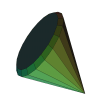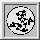Up: Qhull manual: contents
To: ProgramsOptionsOutputFormatsGeomviewPrintQhullPrecisionTraceFunctions (local)
To: synopsis • outputs • examples • notes • options

#rbox -- generate point distributions

rbox generates random or regular points according to the options given, and outputs the points to stdout. The points are generated in a cube, unless 's', 'x', or 'y' are given.

### »rbox synopsis

```rbox- generate various point distributions.  Default is random in cube.

args (any order, space separated):
3000    number of random points in cube, lens, spiral, sphere or grid
D3      dimension 3-d
c       add a unit cube to the output ('c G2.0' sets size)
d       add a unit diamond to the output ('d G2.0' sets size)
l       generate a regular 3-d spiral
r       generate a regular polygon, ('r s Z1 G0.1' makes a cone)
s       generate cospherical points
x       generate random points in simplex, may use 'r' or 'Wn'
y       same as 'x', plus simplex
Cn,r,m  add n nearly coincident points within radius r of m points

Ln      lens distribution of radius n.  Also 's', 'r', 'G', 'W'.
Mn,m,r  lattice (Mesh) rotated by [n,-m,0], [m,n,0], [0,0,r], ...
'27 M1,0,1' is {0,1,2} x {0,1,2} x {0,1,2}.  Try 'M3,4 z'.
W0.1    random distribution within 0.1 of the cube's or sphere's surface
Z0.5 s  random points in a 0.5 disk projected to a sphere
Z0.5 s G0.6 same as Z0.5 within a 0.6 gap

Bn      bounding box coordinates, default 0.5
h       output as homogeneous coordinates for cdd
n       remove command line from the first line of output
On      offset coordinates by n
t       use time as the random number seed (default is command line)
tn      use n as the random number seed
z       print integer coordinates, default 'Bn' is 1e+06
```

### »rbox outputs

The format of the output is the following: first line contains the dimension and a comment, second line contains the number of points, and the following lines contain the points, one point per line. Points are represented by their coordinate values.

For example, rbox c 10 D2 generates

```2 RBOX c 10 D2
14
-0.4999921736307369 -0.3684622117955817
0.2556053225468894 -0.0413498678629751
0.0327672376602583 -0.2810408135699488
-0.452955383763607 0.17886471718444
0.1792964061529342 0.4346928963760779
-0.1164979223315585 0.01941637230982666
0.3309653464993139 -0.4654278894564396
-0.4465383649305798 0.02970019358182344
0.1711493843897706 -0.4923018137852678
-0.1165843490665633 -0.433157762450313
-0.5   -0.5
-0.5    0.5
0.5   -0.5
0.5    0.5
```

### »rbox examples

```       rbox 10
10 random points in the unit cube centered  at  the
origin.

rbox 10 s D2
10 random points on a 2-d circle.

rbox 100 W0
100 random points on the surface of a cube.

rbox 1000 s D4
1000 random points on a 4-d sphere.

rbox c D5 O0.5
a 5-d hypercube with one corner at the origin.

rbox d D10
a 10-d diamond.

rbox x 1000 r W0
100 random points on the surface of a fixed simplex

rbox y D12
a 12-d simplex.

rbox l 10
10 random points along a spiral

rbox l 10 r
10 regular points  along  a  spiral  plus  two  end
points

rbox 1000 L10000 D4 s
1000 random points on the surface of a narrow lens.

rbox 1000 L100000 s G1e-6
1000 random points near the edge of a narrow lens

rbox c G2 d G3
a cube with coordinates +2/-2 and  a  diamond  with
coordinates +3/-3.

rbox 64 M3,4 z
a  rotated,  {0,1,2,3} x {0,1,2,3} x {0,1,2,3} lat-
tice (Mesh) of integer points.

rbox P0 P0 P0 P0 P0
5 copies of the origin in 3-d.  Try 'rbox P0 P0  P0
P0 P0 | qhull QJ'.

r 100 s Z1 G0.1
two  cospherical  100-gons plus another cospherical
point.

100 s Z1
a cone of points.

100 s Z1e-7
a narrow cone of points with many precision errors.
```

### »rbox notes

Some combinations of arguments generate odd results.

### »rbox options

```       n      number of points

Dn     dimension n-d (default 3-d)

Bn     bounding box coordinates (default 0.5)

l      spiral distribution, available only in 3-d

Ln     lens  distribution  of  radius n.  May be used with
's', 'r', 'G', and 'W'.

Mn,m,r lattice  (Mesh)  rotated  by  {[n,-m,0],   [m,n,0],
[0,0,r],  ...}.   Use  'Mm,n'  for a rigid rotation
with r = sqrt(n^2+m^2).  'M1,0'  is  an  orthogonal
lattice.   For  example,  '27  M1,0'  is  {0,1,2} x
{0,1,2} x {0,1,2}.

s      cospherical points randomly generated in a cube and
projected to the unit sphere

x      simplicial  distribution.   It  is fixed for option
'r'.  May be used with 'W'.

y      simplicial distribution plus a simplex.   Both  'x'
and 'y' generate the same points.

Wn     restrict  points  to distance n of the surface of a
sphere or a cube

c      add a unit cube to the output

c Gm   add a cube with all combinations of +m  and  -m  to
the output

d      add a unit diamond to the output.

d Gm   add a diamond made of 0, +m and -m to the output

Cn,r,m add n nearly coincident points within radius r of m points

nates with 0.0.

n      Remove the command line from the first line of out-
put.

On     offset the data by adding n to each coordinate.

t      use  time  in  seconds  as  the  random number seed
(default is command line).

tn     set the random number seed to n.

z      generate integer coordinates.  Use 'Bn'  to  change
the  range.   The  default  is 'B1e6' for six-digit
coordinates.  In R^4, seven-digit coordinates  will
overflow hyperplane normalization.

Zn s   restrict points to a disk about the z+ axis and the
sphere (default Z1.0).  Includes the opposite pole.
'Z1e-6'  generates  degenerate  points under single
precision.

Zn Gm s
same as Zn with an empty center (default G0.5).

r s D2 generate a regular polygon

r s Z1 G0.1
generate a regular cone
```The Geometry Center Home Page